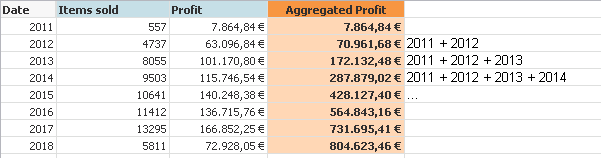# QlikView App Dev

Discussion Board for collaboration related to QlikView App Development.

cancel
Showing results for
Did you mean:Contributor III

## Pivot table with aggregated columns

Hi

I want to create a Pivot Table where I will show Items sold and profit per year (row). In addition, I want to show a third metric column with the aggregated profit from first row until each row. For example, forth row (2014) will be the sum profit of 2011 + 2012 + 2013 + 2014.I'm trying to get it with Set Analisis, AGGR and TOTAL functions, without success. Please, anyone knows how can I get it?

Cheers

1 Solution

Accepted SolutionsMVP

May be try this

RangeSum(Above(Sum(Profit), 0, RowNo()))

6 RepliesMVP

May be try this

RangeSum(Above(Sum(Profit), 0, RowNo()))MVP & Luminary

You need interrecord-functions for this tasks, for example:

rangesum(above(sum(Profit), 0, rowno(total)))

- MarcusContributor III
Author

Thanks!!, it works but the partial sum (default row with total) is not calculated, is it normal?Contributor III
Author

Thanks Marcus!MVP

You might be able to use Dimensionality() function to calculate a partial sum... but I am not sure what the partial sum needs to look like for accumulated value?

How to use - Dimensionality()Contributor III
Author

Thanks, I will check it